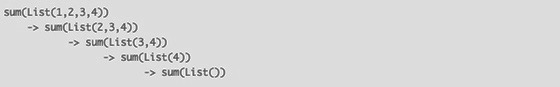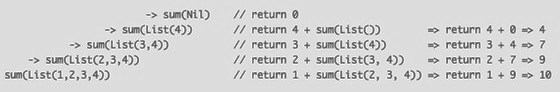# Recursion: How Recursive Function Calls Work

An important point to understand about recursive function calls is that just as they “wind up” as they are called repeatedly, they “unwind” rapidly when the function’s end condition is reached.

In the case of the `sum` function, the end condition is reached when the `Nil` element in a `List` is reached. When `sum` gets to the `Nil` element, this pattern of the `match` expression is matched:

``````case Nil => 0
``````

Because this line simply returns `0`, there are no more recursive calls to `sum`. This is a typical way of ending the recursion when operating on all elements of a `List` in recursive algorithms.

As I wrote in the earlier `List` lesson, a literal way to create a `List` is like this:

``````1 :: 2 :: 3 :: 4 :: Nil
``````

This is a reminder that with any Scala `List` you are guaranteed that the last `List` element is `Nil`. Therefore, if your algorithm is going to operate on the entire list, you should use:

``````case Nil => ???
``````

This is the first clue about how the unfolding process works.

Note 1: This is a feature of the Scala `List` class. You’ll have to change the approach if you work with other sequential collection classes like `Vector`, `ArrayBuffer`, etc. (More on this later in the book.)

Note 2: Examples of functions that work on every element in a list are `map`, `filter`, `foreach`, `sum`, `product`, and many more. Examples of functions that don’t operate on every list element are `take` and `takeWhile`.

A good way to understand how the `sum` function example ran is to add `println` statements inside the `case` expressions.

First, change the `sum` function to look like this:

``````def sum(list: List[Int]): Int = list match {
case Nil => {
println("case1: Nil was matched")
0
}
case head :: tail => {
}
}
``````

Now when you run it again with a `List(1,2,3,4)` as its input parameter, you’ll see this output:

``````case2: head = 1, tail = List(2, 3, 4)
case2: head = 2, tail = List(3, 4)
case2: head = 3, tail = List(4)
case2: head = 4, tail = List()
case1: Nil was matched
``````

That output shows that `sum` is called repeatedly until the list is reduced to `List()` (which is the same as `Nil`). When `List()` is passed to `sum`, the first `case` is matched and the recursive calls to `sum` come to an end. (I’ll demonstrate this visually in the next lesson.)

The book, Land of Lisp states, “recursive functions are list eaters,” and this output shows why that statement is true.

Keeping in mind that `List(1,2,3,4)` is the same as `1::2::3::4::Nil`, you can read the output like this:

1. The first time `sum` is called, the `match` expression sees that the given `List` doesn’t match the `Nil` element, so control flows to the second `case` statement.

2. The second `case` statement matches the `List` pattern, then splits the incoming list of `1::2::3::4::Nil` into (a) a head element of `1` and

1. the remainder of the list, `2::3::4::Nil`. The remainder — the tail — is then passed into another `sum` function call.
3. A new instance of `sum` receives the list `2::3::4::Nil`. It sees that this list does not match the `Nil` element, so control flows to the second `case` statement.

4. That statement matches the `List` pattern, then splits the list into a head element of `2` and a tail of `3::4::Nil`. The tail is passed as an input parameter to another `sum` call.

5. A new instance of `sum` receives the list `3::4::Nil`. This list does not match the `Nil` element, so control passes to the second `case` statement.

6. The list matches the pattern of the second `case` statement, which splits the list into a head element of `3` and a tail of `4::Nil`. The tail is passed as an input parameter to another `sum` call.

7. A new instance of `sum` receives the list `4::Nil`, sees that it does not match `Nil`, and passes control to the second `case` statement.

8. The list matches the pattern of the second `case` statement. The list is split into a head element of `4` a tail of `Nil`. The tail is passed to another `sum` function call.

9. The new instance of `sum` receives `Nil` as an input parameter, and sees that it does match the `Nil` pattern in the first `case` expression. At this point the first `case` expression is evaluated.

10. The first `case` expression returns the value `0`. This marks the end of the recursive calls.

At this point — when the first `case` expression of this `sum` instance returns `0` — all of the recursive calls “unwind” until the very first `sum` instance returns its answer to the code that called it.

That description gives you an idea of how the recursive `sum` function calls work until they reach the end condition. Here’s a description of what happens after the end condition is reached:

1. The last `sum` instance — the one that received `List()` — returns `0`. This happens because `List()` matches `Nil` in the first `case` expression.

2. This returns control to the previous `sum` instance. The second `case` expression of that `sum` function has `return 4 + sum(Nil)` as its return value. This is reduced to `return 4 + 0`, so this instance returns `4`. (I didn’t use a `return` statement in the code, but it’s easier to read this now if I say “return.”)

3. Again, this returns control to the previous `sum` instance. That `sum` instance has `return 3 + sum(List(4))` as the result of its second `case` expression. You just saw that `sum(List(4))` returns `4`, so this `case` expression evaluates to `return 3 + 4`, or `7`.

4. Control is returned to the previous `sum` instance. Its second `case` expression has `return 2 + sum(List(3,4))` as its result. You just saw that `sum(List(3,4))` returns `7`, so this expression evaluates to `return 2 + 7`, or `9`.

5. Finally, control is returned to the original `sum` function call. Its second `case` expression is `return 1 + sum(List(2,3,4))`. You just saw that `sum(List(2,3,4))` returns `9`, so this call is reduced to `return 1 + 9`, or `10`. This value is returned to whatever code called the first `sum` instance.

One way to visualize how the recursive `sum` function calls work — the “going down” part — is shown in Figure [fig:recursionGoingDown].After that, when the end condition is reached, the “coming back up” part — what I call the unwinding process — is shown in Figure [fig:recursionUnwinding].If this isn’t clear, fear not, in the next lesson I’ll show a few more visual examples of how this works.